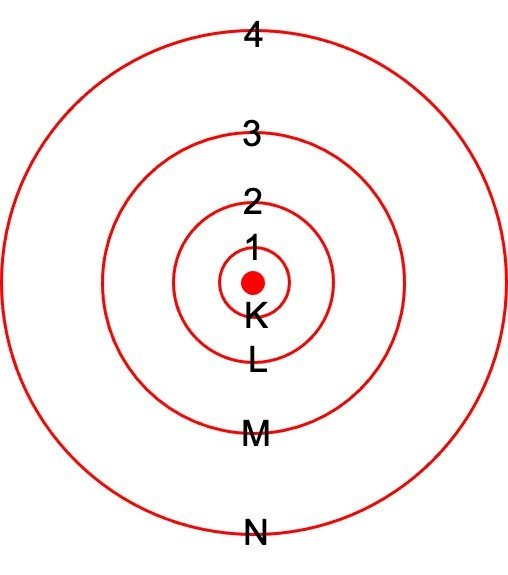Research Tweet

# Bohr Model

Bohr model of the atom was proposed by Neil Bohr in the year 1915. It was an extended modification of Rutherford’s model of an atom. Rutherford made the first discovery by presenting the model of an atom, in which he described that a nucleus (a positively charged particle) is surrounded by an electron which are negatively charged particles. Though this model has some discrepancies or drawbacks, Bohr tried to resolve them in his model.

Bohr with his enhanced version of atomic structure proposed that electrons always move in a fixed path called orbits or energy shells and he also explained that each orbit (or energy shell) possessed a fixed energy level.Rutherford primarily described the nucleus of an atom and Bohr reformed that model into electrons and their energy levels.

## Bohr Model of an Atom

Bohr model atom comprises of a small nucleus (positively charged) surrounded by negatively charged particles termed as electrons which revolve around the nucleus in fixed energy shell called orbits.

Bohr stated that an electron situated away from the nucleus has more energy, as compared to electrons close to the nucleus.

### Postulates of Bohr Model of an Atom

• In an atom, electrons (negatively charged particles) rotate around the positively charged nucleus in a fixed path called orbits or energy shells.

• Each orbit or energy shell has fixed energy.

• The energy levels of the shell are characterized by a simple whole number (that is n=1, 2, 3…) often designated as the quantum number. The orbits n=1, 2, 3, 4… are also known as K, L, M, N…. shells, and when an electron is present at the lowest energy level, it is said to be present in the ground state.

• Electrons present in an atom gains energy when it moves from a lower energy level to a higher energy level, however, if the electron jumps from a higher energy level to lower energy, then the energy is emitted.

• The energy absorbed or emitted is always equal to the difference between the energies of the two given energy levels (that is E1 and E2) and is determined by Plank’s equation. The equation for the same is given below;

ΔE = E2-E1 = hv

Where,

ΔE represents the energy that is absorbed or emitted

h represents Plank’s constant

v represents the frequency of electromagnetic radiation emitted or absorbed

#### Limitations of Bohr Model

• Bohr’s theory of the atomic model was quite effective in explaining the stability of the atom and the line spectrum of a hydrogen atom.

• Orbits were presumed to be circular but Sommerfield further stated that these orbits are elliptical.

• The intensity of spectral lies could not be clarified by Bohr.

• The nucleus was told to be stationary or inactive but it was later revealed that the nucleus (positively charged particle) revolves around its own axis.

• Bohr model of the atom was unable to explain the Zeeman effect (that is splitting up of spectral lines in the magnetic field) and Stark effect (that is splitting up in electric field)

##### Significant Limitations of Bohr Atomic Model

• Bohr was not able to explain the shapes of the molecule due to the directional bonding between atoms. This model has a very incomplete approach in terms of the size of atoms. The Bohr atomic model did not make precise predictions for large-sized atoms but it did provide adequate information for smaller atoms like hydrogen.

• Bohr was unable to explain the line spectra of atoms encompassing of more than one electron thus called as multi-electron atoms. According to Bohr’s model of the atom, one and only one spectral line can be created from an electron between any two energy levels. But by using powerful spectroscopy, it was further observed that certain single lines split into a number of very closely related lines. The existence of such a line could not be explained by Bohr.

• Bohr’s theory failed to explain the effect of the magnetic field on the spectra of atoms or ions. It does not explain the Zeeman Effect that is defined as the splitting of the spectral line into numerous other components in the presence of a magnetic field.

• Bohr’s theory failed to explain the effect of an electric field also termed as the Stark effect on the spectra of atoms. Stark Effect is defined as the splitting of the spectral line into a number of closely related lines in the presence of an electric field.

• The Bohr model violates the Heisenberg Uncertainty Principle because the Bohr Model considers electrons to have both a known radius as well as an orbit, which is not possible according to the Heisenberg principle. Bohr described that an electron in an atom revolves around a nucleus with explicit velocity, which means that it is associated with a fixed value of momentum. This further contradicted the Heisenberg’s Uncertainty Principle.

Thus, we find that Bohr’s model was partially successful. It did describe some of the experimental outcomes but was unable to explain the other features of the atom. Hence, the Bohr model of the atom was soon abandoned in the light of modern ideas relating to the wave characteristics of matter.

##### Refinements and Improvements to the Bohr Model

The most significant improvement in the Bohr model was done by Sommerfeld or the Bohr-Sommerfeld model. In this model, it was discovered that electrons travel in elliptical orbits around the nucleus rather than in circular orbits. The Sommerfeld model was also able to explain atomic spectral effects, such as the Stark effect. Though, the model failed to explain the magnetic quantum number. Eventually, the Bohr model and models based upon it were substituted by Pauli’s model which was based on quantum mechanics.

##### Limitations of Bohr Model Citations

Share

###### Similar Post: